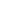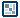## Question of the WeekFinding Maximum/Minimum Value in an Array
by kirupa  |  1 July 2005

I was a bit dismayed to find out that Flash had no simple built-in way of getting the maximum value from an array. You have Math.max(), but that function returns only the greater of two values. The Array.sort and Array.sortOn methods were too complicated for me to fiddle with, and when I thought I had them working, they didn't produce the right results.

In this short tutorial, I will provide the code for finding the maximum and minimum values in an array. I will conclude with a brief explanation of the ideas behind why the code work with an explanation of the code itself.

Code for Finding the Maximum Value in an Array:

maxValue = function (array) {
mxm = array;
for (i=0; i<array.length; i++) {
if (array[i]>mxm) {
mxm = array[i];
}
}
return mxm;
};

Code for Finding the Minimum Value in an Array:

minValue = function (array) {
mn = array;
for (i=0; i<array.length; i++) {
if (array[i]<mn) {
mn = array[i];
}
}
return mn;
};
To use either of the above functions, simply call minValue or maxValue with an array as an argument. For example:
k = new Array();
k = [12, 5, 63, 7, 1, -1, 352, 36, 754, 75, 56, 74];
trace(maxValue(k)); // 754
trace(minValue(k)); // -1

Since the largest number in our k array is 754, our maxValue returns a 754 when traced with k as the argument. Likewise, running the minValue function with k as the argument returns the smallest value, -1.

Thinking About Finding the Max Value in an Array
Before I explain the code, I would like to first talk generically about how to approach a problem such as this. You have an array with a long list of numbers, and your goal is to find the maximum minimum value from that list of numbers. (For simplicity, I will focus only on the maximum case. The minimum case is simply the opposite.)

Here is a more detailed form of our array:In the above image, I added the index positions to our sample array k that I used in my above example.

So now, your job is to start off at the beginning and find out what the maximum value is. Since you are just starting out, you have no real frame of reference. You can't compare whether the number in the zero-th position is a maximum or not, so you automatically set that number as your temporary maximum.

In other words, the 12 at position 0 is the current maximum number. You then move to the next number, position 1, and ask yourself, "Is 5 greater than the current maximum, 12?" The answer is no. 5 is not greater than 12, so twelve still remains your current, local maximum.

You now progress to index position 2. At position 2, the value in your array is a 63. You ask yourself the similar question, "Is 63 greater than the current maximum, 12?". The answer is yes. 63 is greater than 12, so now your new maximum is 63.

You will keep progressing through each index position and asking yourself whether the number at this position is greater than the current maximum. If the number is greater, then you replace your old maximum value with the new number you are at. If the number you are at is not greater, you simply progress to the next number and ask yourself if that number is greater. You keep going until you reach the end of your array.

When you reach the final value in your array, you can throw up your hands and boldly declare that whatever maximum value you have after your last value is the maximum value of your entire array!

For finding the minimum, instead of asking, "Is this number greater than the current maximum?", you would ask, "Is this number less than the current minimum?"

Code Explained
Again, I will focus only on the maxValue function. The code for minValue is exactly the same except for one operator change and a  few variable naming changes.

maxValue = function (array) {

In this line I am declaring a new function called maxValue that takes in an argument. Even though I call my argument 'array', you can pass anything you want into it. If you don't pass an array, though, you will run into problems. The solution to that would be to ensure that users pass in an array with numbers, but it's implementation is something well beyond the scope of this tutorial.

mxm = array;

I declare the variable mxm with an initial value - the first value in our array. If you recall from my earlier explanation, we can safely assume that the first value in our array will be our temporary maximum value.

You may also use a ridiculously small number for the initial maximum value or a large number for the initial minimum value, but you may run into a case where one of the numbers lie beyond your initial value.

For example, if your initial value is a 0 and the largest number in your array is a negative 2, then the initial value will be deemed the maximum value. The problem is that you arbitrarily picked 0 as your initial value. It was never a part of your dataset (array), so that maximum value is invalid.

As long as you keep the initial max/min value as the first value in your array, you should not run into situations where the final result is a number that you made up.

for (i=0; i<array.length; i++) {
if (array[i]>mxm) {
mxm = array[i];
}
}

I initiate a simple loop that counts from 0 until it reaches the end of our array. This is representative of me progressing through each value in our array until I reach the end.

if (array[i]>mxm) {
mxm = array[i];
}

In these lines of code, I first check if the number in our array at position i is greater than our maximum value. If it is, I set our current value to be our new maximum. If our current value is not greater than our current maximum, I just don't do anything.

return mxm;

After all is said and done, and our value of i is less than the length of our array, I return the maximum value, mxm. In short, that is what our function, in the end outputs - just the maximum value as determined by all of our above work.

For the minimum, the only difference is that array[i] > mxm is replaced with array[i] < mn, where mxm and mn are similar variables that store our temporary maximums and minimums.

If you are interested in not only finding the maximum/minimum but also the position at which the maximum/minimum occurs, you may find the following article at Layer 51 useful: http://proto.layer51.com/d.aspx?f=254 Thanks to lostinbeta for providing me with the URL.

Got a question or just want to chat? Comment below or drop by our forums (they are actually the same thing!) where a bunch of the friendliest people you'll ever run into will be happy to help you out!

Get cool tips, tricks, selfies, and more...personally hand-delivered to your inbox!

( View past issues for an idea of what you've been missing out on all this time! )